# Mba studium

At MBA school, fourth-year students can choose from three optional subjects:
a) mathematical methods,
b) social interaction,
c) management

Each student studies one of these subjects. The mathematical methods studied 28 students, the social interaction 27 pupils, and the management 23 pupils. Seven of them simultaneously study mathematical methods and social interaction, six mathematical methods and management, and five social interaction and management. Two pupils attend all three optional subjects. How many pupils are in the fourth year?

Result

n =  56

#### Solution:Leave us a comment of example and its solution (i.e. if it is still somewhat unclear...):Be the first to comment!## Next similar examples:

1. The HotelThe Holiday Hotel has the same number of rooms on each floor. Rooms are numbered with natural numbers sequentially from the first floor, no number is omitted, and each room has a different number. Three tourists arrived at the hotel. The first one was in r
2. Holidays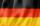Of the 35 students of class were 7 on holiday in Germany and just as much in Italy. 5 students visited Austria. In none of these countries was 21 students, all three visited by one student . In Italy and Austria were 2 students and in Austria and Germany.
3. Three students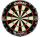Three students independently try to solve the problem. The first student will solve a similar problem with a probability of 0.6, the second student will solve at a probability of 0.55, and the third will solve at a probability 0.04. The problem is resolved
4. Left handedIt is known that 25% of the population is left-handed. What is the probability that there is a maximum of three left-handers at a seminar where there are 30 participants?
5. Intersect and conjuctionLet U={1,2,3,4,5,6} A={1,3,5} B={2,4,6} C={3,6} Find the following. 1. )AUB 2. )A'UB'
6. Two doctorsDoctor A will determine the correct diagnosis with a probability 86% and doctor B with a probability 86%. Calculate probability of correct diagnosis if patient is diagnosed by both doctors.
7. SegmentsLine segments 62 cm and 2.2 dm long we divide into equal parts which lengths in centimeters is expressed integer. How many ways can we divide?
8. Dices throwsWhat is the probability that the two throws of the dice: a) Six falls even once b) Six will fall at least once
9. Utopia IslandA probability of disease A on the island of Utopia is 40%. A probability of occurrence among the men of this island, which make up 60% of all the population (the rest are women), is 50%. What is the probability of occurrence of A disease among women on Uto
10. News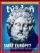From 28 students buy magazine 10 The economist students, 10 Pravda students and 18 students don't buy any of these magazines. How many students buy both magazines?
11. 80 students80 students were asked what type of test they preferred. 50 students said they liked multiple choice and 42 liked true or false. If 36 liked both multiple choice and true or false types, how many students preferred multiple choice only?
12. Glasses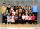Imagine a set of students in your class (number of students: 19), who wears glasses. How much minimum and maximum element may contain this set.
13. Trousers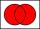In the class was 12 students. Nine students wearing trousers and turtleneck eight. How many students worn trousers with a turtleneck?
14. Class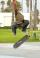The class has 18 students. Everyone knows inline skating or skateboarding. Inline skating can ride 11 students on a skateboard 10. How many ride on inline skates and on skateboard?
15. PupilsThere are 27 pupils in the classroom. They can swim 21 and ski nine pupils. Neither swim nor ski three pupils. How many pupils can swim and ski?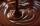In the box were total of 200 cookies. These products have sugar and chocolate topping. Chocolate topping is used on 157 cookies. Sugar topping is used on 100 cakes. How many of these cookies has two frosting?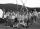In a class are 26 children. During the holidays 16 children were in the camps and 14 children on holiday with their parents. Determine the minimum and maximum number of children that may have been in the camp and on holiday with their parents at the same